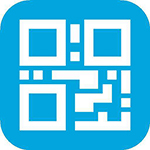# PHP生成带有logo和底图的支付二维码

## 前导：

最近项目中需要做一个二维码图片，来负责将支付宝和微信的支付合并的图片。图片跟大家平常看到的微信或者支付宝中生成的二维码没有什么区别。但是用php的qrcode生成的二维码呢，只是简单的一个二维码，还需要将二维码嵌入到背景图片中，下面就来看一下我最近弄的二维码。## 生成二维码：

png()方法有6个参数：

$QRDir = "./base.png"; //生成的图片路径$errorCorrectionLevel = 'H';//容错率
$matrixPointSize = 10;//生成的图片的大小$margin = 2; 

$qrCode = new QRcode();$qrCode->png($text,$QRDir, $errorCorrectionLevel,$matrixPointSize, $margin); 通过上面的操作，我们就生成了一个二维码，并且将这个二维码的内容保存在了base.png图片中。 如果是简单的要得到一个二维码的话，那么通过上面的操作就达到目的。但是只是单单一个二维码显得有点难看，实际项目中需要将这个二维码进行美化，加入一些我们需要的背景和logo。接下来我们进行二维码进一步加工，将二维码变的美观起来。 我的实际项目中有2种情况，一种是生成一个不带logo的二维码，一种是带logo的二维码。 ## 我们先来说不带logo的二维码的生成： 由于我们生成的二维码不一定能够百分百的放入到我们预先设置好的背景图片中：（当然也可以在制作背景图片的时候测量好尺寸，正好将二维码放入到背景图片中），这里需要解释一下什么是百分百放入到背景图片中，就是生成的二维码跟背景中预留的显示二维码的空白处能够正好合上，由于背景有时候会是变化的，生成的二维码的大小是固定的，这样的话就不能满足我们需求。 遇到上面变化的背景图时，就需要对生成的二维码进行处理，对生成的二维码进行放大或者缩小的操作。 需要简绍几个图像处理的函数： imagecreatefromgif()：创建一块画布，并从 GIF 文件或 URL 地址载入一副图像 imagecreatefromjpeg()：创建一块画布，并从 JPEG 文件或 URL 地址载入一副图像 imagecreatefrompng()：创建一块画布，并从 PNG 文件或 URL 地址载入一副图像 imagecreatefromwbmp()：创建一块画布，并从 WBMP 文件或 URL 地址载入一副图像 imagecreatefromstring()：创建一块画布，并从字符串中的图像流新建一副图像 使用上面的函数，把生成的二维码图片读取出来，获取到二维码的高度跟宽度。 $QRImageInfo = imagecreatefromspng($QRDir);$QR_width = imagesx($QRImageInfo);$QR_height = imagesy($QRImageInfo); 然后再创建一副我们需要大小的图片： 使用imagecreatetruecolor()方法，创建图片资源，使用imagecolorallocate()方法，给图片设置背景色 $width = 539;
$height = 539;$newImage = imagecreatetruecolor($width,$height);//创建一个图像资源
$newImage_white = imagecolorallocate($newImage, 255,255,255);//给创建好的图像资源设置白色背景
imagefill($newImage, 0, 0,$newImage_white);//填充$newImage 接下来使用imagecopyresized()函数将二维码图缩放到新建的图片上： imagecopyresized()函数有10个参数： 1、要放到的新图片资源（目标图片资源）dst_im 2、要缩放的图片资源（原图片资源）src_im 3、目标图像开始x坐标 dst_x 4、目标图像开始y坐标 dst_y 5、原图像的开始x坐标 src_x 6、原图像的开始y坐标 src_y 7、目标图像的宽度 dst_w 8、目标图像的高度 dst_h 9、原图像的宽度 src_w 10、原图像的高度 src_h 根据上面的参数我们就可以将二维码图片放入到新建的图像中了： imagecopyresized($newImage, $QRImageInfo,0, 0, 0, 0, 539, 539,$QR_width, $QR_height); 这样得到的$newImage就是一个缩放了的二维码。

imagecopyresampled这个函数和上面imagecopyresized的效果是一样的，只不过这个函数处理出来的效果要比imagecopyresized好。参数也是一样的。

$backgroundDir = "./background.png";//事先准备好的背景图片$backgroundImg = imagecreatefromspng($backgroundDir); imagecopyresampled($backgroundImg, $newImage, 203, 330, 0, 0, 539, 539, 539, 539); 最后就是将合并好的图片保存起来： $resultPngPic = "./resultPngPicWithoutLogo.png";//设置最后生成图像的路径
imagepng($backgroundImg,$resultPngPic);
imagedestroy($newImage);//销毁中间创建的$newImage资源

## 接下来就是生成带Logo的二维码：$logoDir = "./logo.png";$resource = imagecreatefrompng($logoDir);$image_width = imagesx($resource);$image_height = imagesy($resource); 创建一个跟当前图片相同大小的画布： $targetImg = imagecreatetruecolor($logo_width,$logo_height);
imagesavealpha($targetImg, true);//保留图片的透明通道$targetImgBackground = imagecolorallocatealpha($targetImg, 255, 255, 255, 127);//将目标图片设置成透明背景 imagefill($targetImg, 0, 0, $targetImgBackground);//填充透明背景 接下来就是将logo图片画到目标图片上： 这里有俩个方法，第一种比较灵活，但是效率低：就是判断每一个像素点是否落在了我们我们要的范围内，落在了我们要范围内的话，就将这个像素点画在画布上，重复这一过程，直到所有像素点都遍历完。这样我们就相当于裁剪出一个新的图片。这种方法可以裁剪任何样子的图片，只要你能够做好像素点的判断。但是缺点就是效率太低，需要将所有的像素点遍历一遍，而且需要对画布修改很多次，才能得到最后的图片，如果是专业的需要的话，牺牲点性能也能够接受。但是我们只是需要一个简单的圆角图片。这样的需求完全不需要遍历所有的像素。 第二种方法，就是我们可以创建1个小的正方形，将这个小正形切割成一个扇形，这样讲这个切割后的扇形跟原来的图片合成，就会形成一个圆角图片。但是这里有一个问题，得到的圆角图片只是在形式上是圆角的，圆角的外面是一圈的背景，而不是透明的。 先来看第二种方法： $r = $radius; //圆角半径 function litter_corner($radius){
$img = imagecreatetruecolor($radius, $radius); imagesavealpha($img, true);
$bgcolor = imagecolorallocatealpha($img, 0, 0, 0,127);
$fgcolor = imagecolorallocate($img, 0, 0, 0);
imagefill($img, 0, 0,$bgcolor);
imagefilledarc($img,$radius, $radius,$radius*2, $radius*2, 180, 270,$fgcolor, IMG_ARC_PIE);
imagecolortransparent($img,$fgcolor);
return $img; } // lt(左上角)$lt_corner = litter_corner($radius); imagecopymerge($resource, $lt_corner, 0, 0, 0, 0,$radius, $radius, 100); // lb(左下角)$lb_corner = imagerotate($lt_corner, 90, 0); imagecopymerge($resource, $lb_corner, 0,$image_height - $radius, 0, 0,$radius, $radius, 100); // rb(右上角)$rb_corner  = imagerotate($lt_corner, 180, 0); imagecopymerge($resource, $rb_corner,$image_width - $radius,$image_height - $radius, 0, 0,$radius, $radius, 100); // rt(右下角)$rt_corner  = imagerotate($lt_corner, 270, 0); imagecopymerge($resource, $rt_corner,$image_width - $radius, 0, 0, 0,$radius, $radius, 100);$image = "logoFinal.png";
imagepng($resource,$image);

function radius_img($imgpath,$radius = 15) {
$ext = pathinfo($imgpath);
$src_img = null; switch ($ext['extension']) {
case 'jpg':
$src_img = imagecreatefromjpeg($imgpath);
break;
case 'png':
$src_img = imagecreatefrompng($imgpath);
break;
}
$wh = getimagesize($imgpath);
$w =$wh;
$h =$wh;
// $radius =$radius == 0 ? (min($w,$h) / 2) : $radius;$img = imagecreatetruecolor($w,$h);
//这一句一定要有
imagesavealpha($img, true); //拾取一个完全透明的颜色,最后一个参数127为全透明$bg = imagecolorallocatealpha($img, 255, 255, 255, 127); imagefill($img, 0, 0, $bg);$r = $radius; //圆 角半径 for ($x = 0; $x <$w; $x++) { for ($y = 0; $y <$h; $y++) {$rgbColor = imagecolorat($src_img,$x, $y); if (($x >= $radius &&$x <= ($w -$radius)) || ($y >=$radius && $y <= ($h - $radius))) { //不在四角的范围内,直接画 imagesetpixel($img, $x,$y, $rgbColor); } else { //在四角的范围内选择画 //上左$y_x = $r; //圆心X坐标$y_y = $r; //圆心Y坐标 if (((($x - $y_x) * ($x - $y_x) + ($y - $y_y) * ($y - $y_y)) <= ($r * $r))) { imagesetpixel($img, $x,$y, $rgbColor); } //上右$y_x = $w -$r; //圆心X坐标
$y_y =$r; //圆心Y坐标
if (((($x -$y_x) * ($x -$y_x) + ($y -$y_y) * ($y -$y_y)) <= ($r *$r))) {
imagesetpixel($img,$x, $y,$rgbColor);
}
//下左
$y_x =$r; //圆心X坐标
$y_y =$h - $r; //圆心Y坐标 if (((($x - $y_x) * ($x - $y_x) + ($y - $y_y) * ($y - $y_y)) <= ($r * $r))) { imagesetpixel($img, $x,$y, $rgbColor); } //下右$y_x = $w -$r; //圆心X坐标
$y_y =$h - $r; //圆心Y坐标 if (((($x - $y_x) * ($x - $y_x) + ($y - $y_y) * ($y - $y_y)) <= ($r * $r))) { imagesetpixel($img, $x,$y, $rgbColor); } } } } return$img;
}
$final_logo = radius_img($resource);
imagepng($final_logo, "logoFinal.png"); 通过调用这个函数，我们就能轻松的得到一个透明的圆角logo了，其实也是很简单的，只不过相对来说要比上面的合成图像要繁琐点。 最后呢就是将这个已经修改好的logo图片贴在二维码中间。这里面可能会涉及到图片的大小问题，可以根据之前图片的缩放等办法来得到一个理想的大小。 合成代码如下： $QR = imagecreatefrompng("qr.png");//这里qr.png是二维码的图片
$logo = imagecreatefrompng("logoFinal.png");//圆角二维码logo$QR_width = imagesx($QR);//二维码图片宽度$QR_height = imagesy($QR);//二维码图片高度$logo_width = imagesx($logo);//logo图片宽度$logo_height = imagesy($logo);//logo图片高度$logo_qr_width = $QR_width / 5;$scale = $logo_width/$logo_qr_width;
$logo_qr_height =$logo_height/$scale;$from_width = ($QR_width -$logo_qr_width) / 2;
//重新组合图片并调整大小
imagecopyresampled($QR,$logo, $from_width,$from_width, 0, 0, $logo_qr_width,$logo_qr_height, $logo_width,$logo_height);
$QRHasLogo = "QRHasLogo.png";//最终得到的带有logo的二维码 imagepng($QR, \$QRHasLogo);05-30426
09-26599
09-273191
04-064392
11-242万+
06-05783
07-0630
04-20542
10-261762
06-191969
04-071282
04-105449
11-271万+
04-277516
12-13263
01-1983
©️2020 CSDN 皮肤主题: 编程工作室 设计师:CSDN官方博客